Computational & Technology Resources
an online resource for computational,
engineering & technology publications
Civil-Comp Proceedings
ISSN 1759-3433
CCP: 79
PROCEEDINGS OF THE SEVENTH INTERNATIONAL CONFERENCE ON COMPUTATIONAL STRUCTURES TECHNOLOGY
Edited by: B.H.V. Topping and C.A. Mota Soares
Paper 229

A Jacobi-Davidson Type Projection Method for General Nonlinear Eigenproblems

H. Voss

Section of Mathematics, Hamburg University of Technology, Germany

Full Bibliographic Reference for this paper
H. Voss, "A Jacobi-Davidson Type Projection Method for General Nonlinear Eigenproblems", in B.H.V. Topping, C.A. Mota Soares, (Editors), "Proceedings of the Seventh International Conference on Computational Structures Technology", Civil-Comp Press, Stirlingshire, UK, Paper 229, 2004. doi:10.4203/ccp.79.229
Keywords: eigenvalue, eigenvector, nonlinear eigenproblem, iterative projection method, Jacobi-Davidson method, rational eigenproblem, damped vibrations of structures.

Summary
In this paper we consider the nonlinear eigenvalue problem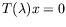(65)

where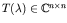is a family of large and sparse matrices depending on a parameter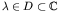. Problems of this type arise in damped vibrations of structures, free vibrations of plates with elastically attached masses, and of rotating structures, stability of linear systems with retarded argument, lateral buckling problems or vibrations of fluid-solid structures, to name just a few. As in the linear case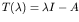a parameter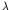is called an eigenvalue of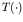if problem (65) has a nontrivial solution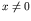which is called a corresponding eigenvector.

For linear sparse eigenproblems iterative projection methods such as the Lanczos, Arnoldi, rational Krylov or Jacobi-Davidson methods are very efficient. In these approaches one determines approximations to the wanted eigenvalues and corresponding eigenvectors from projections of the large eigenproblem to low-dimensional subspaces which are expanded in the course of the algorithm. The small projected eigenproblems are solved by standard techniques.

In some sense, Ruhe  generalised the rational Krylov approach to sparse nonlinear eigenvalue problems by nesting the linearisation of problem (65) by Regula falsi and the solution of the resulting linear eigenproblem by Arnoldi's method, where the Regula falsi iteration and the Arnoldi recursion are knit together. Similarly as in the rational Krylov process he constructs a sequence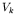of subspaces of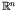, and at the same time he updates Hessenberg matrices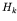which approximate the projection of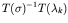to. Here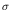denotes a shift and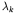an approximation to the wanted eigenvalue of (65). Then a Ritz vector ofcorresponding to an eigenvalue of small modulus approximates an eigenvector of the nonlinear problem from which a (hopefully) improved eigenvalue approximation of problem (65) is obtained. Hence, in this approach the two numerical subtasks, reducing the large dimension to a much smaller one, and solving the projected nonlinear eigenproblems are attacked simultaneously.

In this paper we suggest an iterative projection method for the nonlinear eigenproblem where the two subtasks mentioned in the last paragraph are handled separately. We order the eigenvalues in some way and determine them one after another. If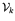denotes the subspace of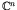of small dimension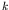constructed in the course of the algorithm, andis an orthonormal basis of, we solve the projected nonlinear eigenvalue problem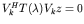of dimensionby a dense solver to obtain approximationsand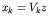to an eigenvalue and eigenvector, respectively. Thereafter we expand the search spaceto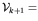span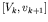and repeat the projection step. Similarly as in the Jacobi-Davidson method for linear eigenproblems the expansion direction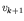ofis chosen such that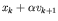for somehas a high approximation potential for the eigenvector we are just aiming at. The projection step and the expansion step are repeated alternately until convergence.

Inverse iteration with variable shifts converges quadratically to simple eigenvalues. Therefore, the expansion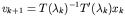would be a reasonable choice. However, in this case we would have to solve a high dimensional linear system in every iteration step where the coefficient matrix varies. A way out is to replace this expansion of the search space by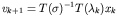suggested by residual inverse iteration. This yields to the nonlinear Arnoldi method proposed in .

For linear eigenproblems it is well known that the Jacobi-Davidson method yields the same expansion of the search space as inverse iteration if the the correction equation is solved exactly, and solving it only approximately still yields fast convergence. The same holds true for nonlinear eigenproblems if the search spaceis expanded by the solution of the correction equation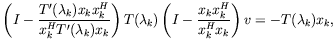(66)

and as in the linear case this equation has to be solved only approximately in a Jacobi-Davidson like manner. For a symmetric problem allowing a minmax characterisation of its eigenvalues this was discussed in . The present paper deals with the general case.

References
1
A. Ruhe. "A rational Krylov algorithm for nonlinear matrix eigenvalue problems", Zapiski Nauchnyh Seminarov POMI, 268, 176 - 180, 2000.
2
H. Voss. "An Arnoldi method for nonlinear eigenvalue problems", Technical Report 56, Section of Mathematics, Hamburg University of Technology, 2002. To appear in BIT Numerical Mathematics.
3
T. Betcke, H. Voss. "A Jacobi-Davidson-type projection method for nonlinear eigenvalue problems", Future Generation Computer Systems, 20, 363 - 372, 2004. doi:10.1016/j.future.2003.07.003

purchase the full-text of this paper (price £20)